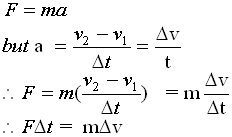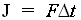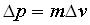#### Impulse & Momentum

Momentum is related to force.  An object with mass m, gains or loses momentum, depending on the direction of the forse, when a force acts on it for a very brief period of time

Mathematically from Newton's Second Law• The left side of the above  equation is known as the impulse (symbol "J").
• The impulse of an object can be described as the force (that caused the object to change its state of motion) acting on the object for a given period of time.
• Note that impulse is a vector quantity.
• Thus one must also take into account its direction as well as its magnitude.The unit of j  is the N.s

• The right side of the equation is known as the change in momentum.
• The change in momentum of an object is given the symbol ∆p.
• Momentum is also a vector quantity and can be defined as the mass-velocity product.
• Momentum is sometimes used as an indicator for the inertia of an object.The unit of ∆p  is the Kg.m/s

In general, for an object in motion, we can say the the impulse is equal to the change in momentum.